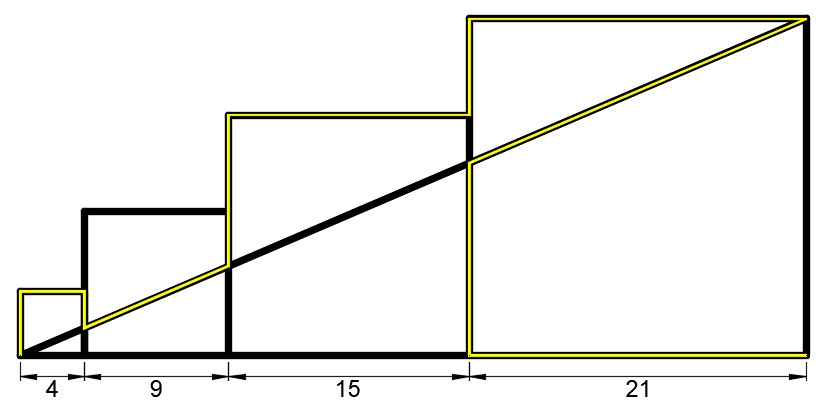# length of the yellow line

Four squares of respective side lengths 4, 9,15, and 21 are arranged as shown. Find the length of the yellow line. If your answer can be expressed as $\frac{a}{7}+\frac{b}{7}\sqrt{c}$, where $c$ is square free, give $a+b+c.$Note: Neglect the thickness of the lines.

×